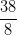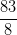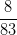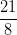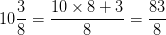# SSAT Upper Level Math : How to find out an improper fraction from a mixed fraction

## Example Questions

← Previous 1

### Example Question #1 : How To Find Out An Improper Fraction From A Mixed Fraction

If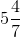is rewritten as an improper fraction, what will its numerator be?Explanation:

The numerator of the improper form of a mixed fraction is the original numerator added to the product of the integer and the original denominator: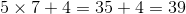### Example Question #2 : How To Find Out An Improper Fraction From A Mixed Fraction

Rewrite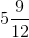as an improper fraction in lowest terms, and callthe sum of its numerator and its denominator. Which of the following is a true statement?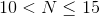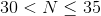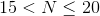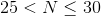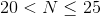Explanation: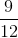, in lowest terms, is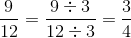, sois rewritten as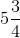.

The numerator of the improper form of a mixed fraction is the original numerator added to the product of the integer and the original denominator. The new denominator is the same as the old one. Therefore,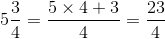,

Add the numerator and the denominator: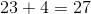.

The correct response is.

### Example Question #3 : How To Find Out An Improper Fraction From A Mixed Fraction

Rewrite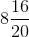as an improper fraction in lowest terms. If we assign tothe numerator of this fraction, which of the following will be a true statement?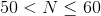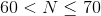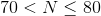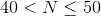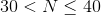Explanation: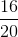in lowest terms is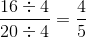, so first, rewriteas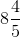.

The numerator of the improper form of a mixed fraction is the original numerator added to the product of the integer and the original denominator: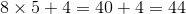The correct response is.

### Example Question #4 : How To Find Out An Improper Fraction From A Mixed Fraction

Rewrite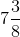as an improper fraction in lowest terms. What will the sum of its numerator and its denominator be?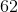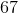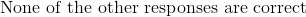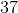Explanation:

The numerator of the improper form of a mixed fraction is the original numerator added to the product of the integer and the original denominator. The new denominator is the same as the old one. Therefore,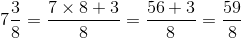Add the numerator and the denominator: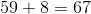### Example Question #5 : How To Find Out An Improper Fraction From A Mixed Fraction

Change the following mixed number into an improper fraction in simplest form: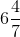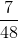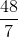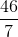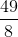Explanation:

To change a mixed fraction into an improper fraction, keep the denominator the same.

To find the numerator, multiply the whole number part of the mixed fraction by the denominator, then add that value to the numerator.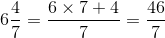### Example Question #6 : How To Find Out An Improper Fraction From A Mixed Fraction

Change the following fraction from a mixed number into an improper fraction in simplest form: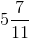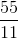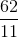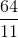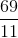Explanation:

To change a mixed number into an improper fraction, keep the denominator. Multiply the whole number part of the mixed number by the denominator and add your result to the given numerator to find the numerator of the improper fraction.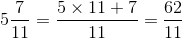### Example Question #7 : How To Find Out An Improper Fraction From A Mixed Fraction

Write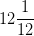as an improper fraction.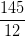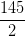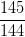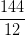Explanation:

To change a mixed number to an improper fraction, keep the denominator.

Then, multiply the whole number by the denominator and add that to the given numerator.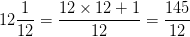### Example Question #8 : How To Find Out An Improper Fraction From A Mixed Fraction

Write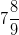as an improper fraction.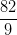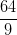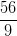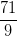Explanation:

To change a mixed number to an improper fraction, keep the denominator.

Then, multiply the whole number by the denominator and add that to the given numerator.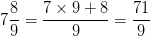### Example Question #1351 : Ssat Upper Level Quantitative (Math)

Write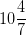as an improper fraction.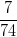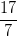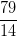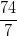Explanation:

To change a mixed number to an improper fraction, keep the denominator.

Then, multiply the whole number by the denominator and add that to the given numerator.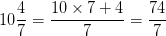### Example Question #10 : How To Find Out An Improper Fraction From A Mixed Fraction

Write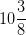as an improper fraction.